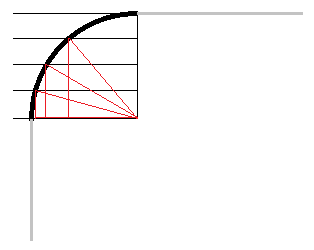## Useful Scripts Collection: Rounded Corners with 2 Lines of JavaScript (No Images!)

Category: Tech
Posted by Jonathan Corbett on September 19th, 2009

The Web 2.0 rounded corner look is popular, and is a frequent request of our clients. Most solutions involve either images that you place in each corner of the containing "divs" to simulate the border being curved, or extremely long and complicated JavaScripts.

We don't like either approach: having to create images each time you want different look is a pain and a waste of bandwidth to download. We also don't like loading up pages with long and convoluted JavaScript; if we can't understand the JavaScript, we don't want to use it.

Therefore, we took an hour and a Red Bull and came up with a JavaScript function to round corners in just 2 lines of code (one is a bit long, but not as complex as it looks):

```function FourTenCorners(theDiv, theWidth, howRound, bgColor){
theDiv.style.backgroundColor = 'transparent';
for(var x = 0; x <= howRound; x++) theDiv.innerHTML = '<div style="width:' + ((theWidth - (2 * howRound)) + (2 * (Math.sqrt((howRound * howRound) - (x * x))))) + 'px;height:' + (x == 0 ? '100%;padding:' + theDiv.style.padding.replace('px', '') : '1px') + ';background-color:' + bgColor + ';margin-left:auto;margin-right:auto;">' + (x == 0 ? '' : '</div>') + theDiv.innerHTML + (x == 0 ? '</div>' : '<div style="width:' + ((theWidth - (2 * howRound)) + (2 * (Math.sqrt((howRound * howRound) - (x * x))))) + 'px;height:' + (x == 0 ? '100%' : '1px') + ';background-color:' + bgColor + ';margin-left:auto;margin-right:auto;">');
}```

The premise is that by creating smaller divs of 1 pixel in height centered on the top and bottom of the original div, rounded corners appear. Visually, let's use a little ASCII-art and say your original div looked like this:
```
XXXXXXXXXXXXXX
X            X
XXXXXXXXXXXXXX

```
Imagine the X's to be the border of your div. If we surrounded the dots with our own, slightly narrower border (represented by Y's) and make the original top and bottom borders transparent, we get:
```
YYYYYYYYYYYY
X            X
X            X
X            X
YYYYYYYYYYYY

```
...which you can see already starts to create a more rounded look. [Note: Math talk follows... avoid unless you really want to know. :)]This is done repeatedly -- the number of times specified in the howRound property, which is essentally the radius of the circle that would fit in your corner. Using that radius, we can calculate the exact width between the border at each pixel by thinking of the circle as having an inscribed right triangle in the visible quadrant (the quarter of the circle that makes up the corner), and use the pythagoream theorum with known hypotenuse and leg.

The image to the right illustrates how the script would calculate the appropriate div width for a radius of 4 on the top left corner of an affected div. The bottom leg of each triangle is the distance sought; the left leg has the known height of 1, 2, and 3 in each respective triangle, and the hypotenuse is the radius, which must always be, in this case, 4.

The function takes 4 parameters: a reference to the div to be modified, the width of the div (in pixels only), the radius of the circle of the corner (integer values only), and the background color of the div (the script doesn't support background images, but if you're going to use a background image rather than a color, you may as well draw your corners in rather than using JavaScript).

Blog Posts by Month